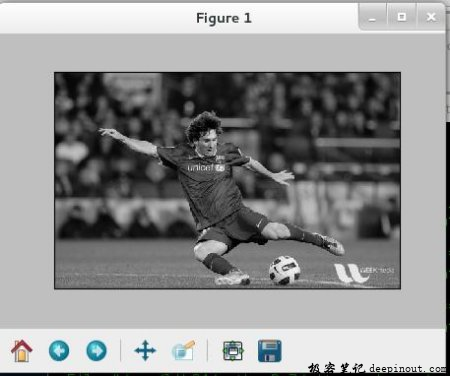# OpenCV-Python图像入门

• 在这里，你将学习如何读取图像、如何显示图像以及如何将其保存起来
• 您还可以选择学习如何使用 Matplotlib 显示图像。

## 使用 OpenCV

### 读取图像

Note

• 你可以简单地分别传递整数 1、0 或-1，而不是这三个 flag。

import numpy as np
import cv2 as cv
# 用灰度模式加载图像


### 显示图像

cv.imshow() 函数在窗口中显示图像，窗口自动适应图像的大小。

cv.imshow('image', img)
cv.waitKey(0)
cv.destroyAllWindows()cv.waitKey() 是一个键盘绑定函数，它的参数是以毫秒为单位的时间。该函数为任意键盘事件等待指定毫秒。如果你在这段时间内按下任意键，程序将继续。如果传的是 0，它会一直等待键盘按下。它也可以设置检测特定的击键，例如，按下键 a 等，我们将在下面讨论。

Note

• 除了绑定键盘事件，该函数还会处理许多其他 GUI 事件，因此你必须用它来实际显示图像。

cv.destroyAllWindows() 简单的销毁我们创建的所有窗口。如果你想销毁任意指定窗口，应该使用函数 cv.destroyWindow() 参数是确切的窗口名。

Note

cv.namedWindow('image', cv.WINDOW_NORMAL)
cv.imshow('image',img)
cv.waitKey(0)
cv.destroyAllWindows()


### 保存图像

cv.imwrite('messigray.png',img)


### 总结一下

import numpy as np
import cv2 as cv

cv.imshow('image',img)
k = cv.waitKey(0)
if k == 27: # ESC 退出
cv.destroyAllWindows()
elif k == ord('s'): # 's' 保存退出
cv.imwrite('messigray.png',img)
cv.destroyAllWindows()


## 使用 Matplotlib

Matplotlib 是一个 Python 的绘图库，提供了丰富多样的绘图函数。你将在接下来的文章中看到它们。在这里，你将学习如何使用 Matplotlib 来显示图像。你还能用 Matplotlib 缩放图像，保存图像等。

import numpy as np
import cv2 as cv
from matplotlib import pyplot as plt

plt.imshow(img, cmap = 'gray', interpolation = 'bicubic')
plt.xticks([]), plt.yticks([]) # 隐藏 X 和 Y 轴的刻度值
plt.show()Matplotlib 提供了大量的绘图选项。有关更多详情信息，请参阅 Matplotlib 文档。有一些，我们用这种方式将会知道。

• 回顶